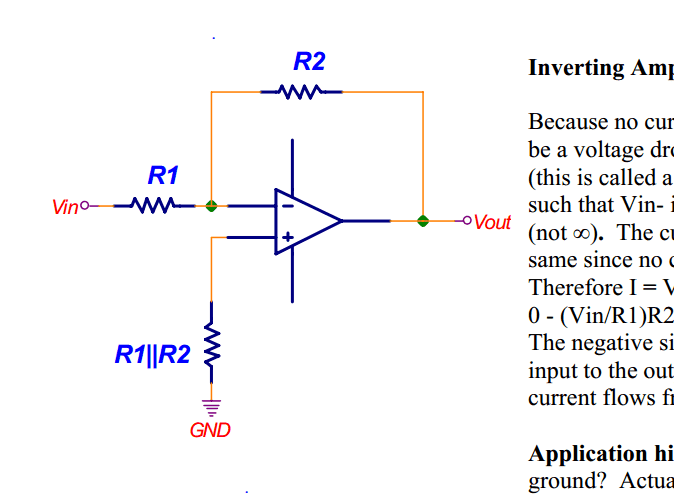# What does it mean physically to apply a voltage to a MOSFET

• LongApple

#### LongApple

https://en.wikipedia.org/wiki/MOSFET

Likewise, the "oxide" in the name can be a misnomer, as different dielectric materials are used with the aim of obtaining strong channels with smaller applied voltages.

http://www.electronics-tutorials.ws/transistor/tran_7.html
In the saturation or linear region, the transistor will be biased so that the maximum amount of gate voltage is applied to the device which results in the channel resistance RDS(on being as small as possible with maximum drain current flowing through the MOSFET switch. Therefore the enhancement type MOSFET is switched “Fully-ON”.

Spiel:

Voltage is kind of like height. I don't have a good analogy of what it would mean to apply height to a terminal. And height requires two points to have height in between. So when we apply a voltage to a switch in a circuit, what are the two points exactly? Voltage at the terminal location to ground?

A mosfet, physically, is a box with three wires sticking out of it - commercial boxes call these "pins".
You are quite right: the phrase "apply a voltage across a mosfet" does not mean anything by itself - you have to use the context to figure out what the voltage is with respect to.

In your quote "the transistor will be biased so that..." just means that the "relative voltages at the pins will be arranged so that...". The "gate voltage" will be indicated on a circuit diagram - usually measured with respect to the circuit's ground rail, the negative side of the battery, something like that.

Have you done any basic transistor or (maybe) linear network theory?

A mosfet, physically, is a box with three wires sticking out of it - commercial boxes call these "pins".
You are quite right: the phrase "apply a voltage across a mosfet" does not mean anything by itself - you have to use the context to figure out what the voltage is with respect to.

In your quote "the transistor will be biased so that..." just means that the "relative voltages at the pins will be arranged so that...". The "gate voltage" will be indicated on a circuit diagram - usually measured with respect to the circuit's ground rail, the negative side of the battery, something like that.

Have you done any basic transistor or (maybe) linear network theory?

no.

"You are quite right: the phrase "apply a voltage across a mosfet" does not mean anything by itself - you have to use the context to figure out what the voltage is with respect to."

How do you know what the voltage is respect to?

Take this example. Is the voltage applied at the negative terminal the voltage between the op amp and the nearest node? Is it the voltage between the op amp and ground? The voltage between the op amp and V in?

http://www.physics.unlv.edu/~bill/PHYS483/op-amps.pdfUse the text that accompanies the diagram... what makes sense? (This looks like a textbook, so you should also bear in mind how the earlier parts of the chapter, and the book, are written.)

In your example, Vin and Vout are measured wrt GND.

This is an exercize in English comprehension: reading technical documents.
It gets harder the higher your education.

Last edited: